Applied and Computational Mathematics
Volume 4, Issue 3, June 2015, Pages: 220-224

Ill-Posed Algebraic Systems with Noise Data

Vladimir V. Ternovski1, Mikhail M. Khapaev1, Alexander S. Grushicin2

1Numerical Math and Cyber Departament, LomonosovStateUniversity, Moscow, Russia

2Information Systems Department, MATI Russian State Technological University, Moscow, Russia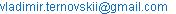(V. V. Ternovski)

Vladimir V. Ternovski, Mikhail M. Khapaev, Alexander S. Grushicin. Ill-Posed Algebraic Systems with Noise Data. Applied and Computational Mathematics. Vol. 4, No. 3, 2015, pp. 220-224. doi: 10.11648/j.acm.20150403.25

Abstract: Finding a numerical solution of linear algebraic equations is known to present an ill-posed in the sense that small perturbation in the right hand side may lead to large errors in the solution. It is important to verify the accuracy of an approximate solution by taking into account all possible errors in the elements of the matrix, and of the vector at the right hand side as well as roundoff errors. There may be computational difficulties with ill-posed systems as well. If to apply standard methods such as the method of Gauss elimination to such systems it may be not possible to obtain the correct solution though discrepancy can be less accuracy of data errors. Besides, a small discrepancy will not always guarantee proximity to a correct solution. Actually there is no need for preliminary assessment whether a given system of linear algebraic equations is inherently ill-conditioned or well-conditioned. In this paper we consider a new approach to the solution of algebraic systems, which is based on statistical effect in matrices of big order. It will be shown that the conditionality of the systems of equation may change with a high probability, if the matrix distorted by random noise. After applying some standard methods, we may introduce the received "chaotic" solution is used as a source of a priori information a more general variational problem.

Keywords: Ill-Posed Problems, Condition Numbers, Random Matrix

1. Introduction

Many traditional direct and iterative traditional numerical methods for solving well-conditioned linear algebraic equations are known. However, these methods tend to be unstable if they are applied for solving ill-conditioned systems. The less known fact is that the commonly used methods of regularization of ill-conditioned problems can be applied to classical methods. We are going to describe this further.

Let A be a square matrix that is ill-conditioned, and consider a system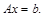(1)

Solving (1) can be a difficult task [1,2,3]. At first, we have to answer on the question: when is a matrix A ill-conditioned? Or, maybe, this question should be better rephrased as: are there any robust condition number estimators for A? It should be noted that the number of elements of a matrix may be very large, making it difficult to investigate system (1) on conditionality, because of the loss of calculations accuracy and significant computation time.

Moreover, we can change value of spectral condition number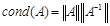(2)

because of scalable system (1).

But it would be problematic to use the condition number as the criterion of quality of ill-conditioned system because of computational difficulties. Besides, complexity of calculation in (2) depends on an assessment of norm of the inverse matrix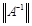, which may result in computational errors. Thus, it makes sense to use the number (2) only if it can be really computed with a fixed accuracy. The above definition of condition number was exposed to criticism in literature, while other options of condition number were discussed in  and other papers.

At the current stage of technological progress, the systems of the linear equations can be conveniently solved via commercial software like MATLAB, MATHEMATICA, MAPLE, etc. Popularity of such software is explained from its ability to carry out calculations both on a personal computer and on a supercomputer, allowing parallelization of calculations with controlled accuracy .

On the other side, there are many systems of linear equations that can’t be solved with the help of commercial software, which gives for them irregular, "chaotic" solutions. The question is about whether it is possible to consider (1) as an ill-conditioned problem, as far as it is not solvable by standard methods of linear algebra? Or, in other words, whether it is possible to consider the system as ill-conditioned, without calculating its condition number? It would be also interesting to know, whether it is possible to avoid calculating the condition number at all.

In further research on such systems, authors propose a new approach that doesn’t require calculation of the system conditionality. This approach is based on statistical phenomena of spectral properties of the perturbed matrix.

If the system (1) is not well-conditioned, the concept of the solution has to be revised, if necessary, by considering the errors of b and A. That is, if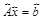, but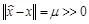, where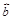is the perturbed value of right hand side vector,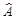- perturbed matrix ,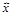is the perturbed solution, x is the original solution, and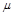is the residual error. The inversion of the perturbed matrix can be correctly calculated because the perturbed matrix is well-conditioned as it will be shown below. However, without using some additional tricks, perturbing the solution is useless in classic approach. At this point a general statement can be made: if the matrix (operator) of A-1 does not exist, the inverse perturbed matrix exists with a high probability. A. N. Tikhonov and others [1,2,6] developed a theory of such ill-posed problems. The focus of his method is to choose the compact class and find the solution with minimal norm, taking into account the errors in the input data.

It should be noted that the relevance of the task of solving ill-conditioned systems does not raise doubts: as such systems occur in numerous engineering applications: recovery of images, spectral analysis, digital signal processing, etc. As we mentioned, solutions obtained using standard programs of computer mathematics can be chaotic. We can also try to get the solution by variational algorithms, but their implementation takes much more time than standard methods of Gauss elimination[1,2]. Again the question remains on whether the obtained solution is meaningful or not.

Now, let us consider a textbook example of solving the algebraic system with Hilbert's matrix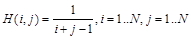.

We define a vector in the right hand side for function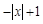on interval from -1 to +1, multiplying a matrix H by the vector with components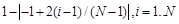. For dimension N=400 calculations the explicit Linear Solve program of the Mathematica package demonstrates instability of the solution. To get the stable solution, it is required to keep 560 digits at least.

From this example, we can draw a conclusion that standard programs may provide reliable solutions only in a case when the elements of the matrix and the vector in the right hand side are known precisely, or when they can be found with controlled accuracy.

Observe that using of Least Squares (built-in Wolfram function ) method gives us a stable solution of the problem with Hilbert matrix, when N=2000 and greater. However, the Least Squares method could be efficient as far as there are no errors in the right hand side. If we perturb the right hand side following a hypothesis about the additivity of errors, the known methods of computational mathematics usually give us unstable solutions and are therefore unsuitable. Least Squares and the pseudo-inverse methods also lead to the irregular solution. Thus, there are the main questions to answer in this paper:

1. How to calculate correct value of the condition number?

2. How to get the correct solution of an ill-conditioned by using standard programs of computer mathematics?

3. How to increase the accuracy of the classical solution of system of the algebraic equations?

The idea of the present article is that an approximate solution obtained via a classical method could be used to solve the associated variation problem. Our assumption is based on the fact that classical solutions, even chaotic, nevertheless are a source of a priori information and they can form a set of possible solutions.

2. Conditionality of System with the Noisy Matrix

Consider a general problem of solving an ill-conditioned system of linear equations when the right hand side and the matrix of the system are subjected to a perturbation. Observe that the spectral properties of the matrix tend to improve as the condition number of an ill-conditioned noisy system decreases with the higher noise amplitude . Thus the condition number of a real-valued random matrix increases as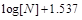, where N - dimension of a matrix . In Fig. 1a the calculated condition numbers of a random matrix are displayed, from where we can observe that these numbers with a high probability do not reach critical values.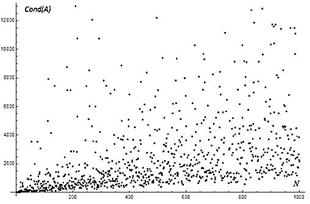Fig. 1a. The condition number of pseudorandom NxN matrices.

According to the theoretical results of  the norm of the inverse noise contaminated matrix in an ill-posed problem and its condition number can be easily calculated. A an example, Fig. 1b shows dependence of the Hilbert matrix condition number on the noise amplitude in the interval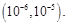The tolerance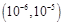is standard for physical experiment errors.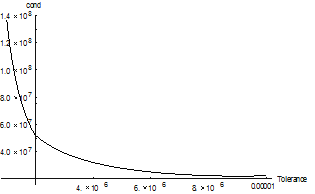Fig. 1b. Condition number of Hilbert Matrix of size1000x1000 is under small perturbation.

These calculations show that with a high probability an ill-conditioned system becomes well-conditioned under a perturbation. Expression "with a high probability" means that we have to repeat perturbation in the case of violation of Fig 1b dependence. Since noise is always present at measurements, it represents a natural addition to the exact matrix and improves the system conditionality. After perturbing the right hand side of the system in the previous example with the Hilbert matrix, with noise amplitude 10-7, the Linear Solve (Mathematica function) method gives us an irregular solution. Other methods also yield the results indicating on chaotic, irregular properties of the solution. Nevertheless, the residual error of such solutionindicates that all calculations are made keeping accuracy. Thus, we may claim the solution to be "chaotic", but in reality, it is the exact classical solution of the perturbed system. Such solution cannot satisfy us though, as according to the results , the system has to be well-conditioned with high probability. We will notice that the methods of smoothing do not reveal the hidden solution if they don't use a priori information about errors. Thus it is necessary to correct the obtained solution within our knowledge on errors. We will note again that the noise contamination of a matrix is made forcibly for the purpose of improvement of conditionality.

There are also exist other effective ways to reduce condition number. For example, it makes sense to consider the extremal problem of finding the condition number of a matrixon a set of diagonal matrices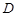with fixed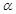and constraint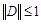. In this case, the perturbed solution can lose physical meaning.

3. The Variational Algorithm

Let us consider a perturbed system, where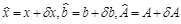, and then let us add a vector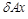to the right and at the left sides of the original system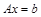, and subtract the perturbed system. This gives us an inequality(3)

where- is the solution of a classical method like Gauss elimination,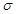- is the error value in the right hand side,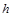- is the maximal absolute value of the matrix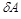.

Finally, consider a variation problem of conditional minimization for solution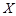: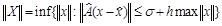(4)

where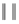is a finite-dimensional norm, such as Frobenius norm, for example.

We now claim that the problem (4) is not the same as original Tikhonov problem , because here, instead ofwe use the solution, that is correctly calculated from the perturbed systemwhich a well-conditioned with a high probability via Gauss elimination or other known methods.

Now, let us consider another problem, when there is a matrix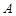(perhaps already perturbed, but errors are unknown), and the right hand side is known only approximately.

In other words, let be the equation,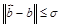, where ill-conditioned,are known, but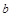is unknown. By using the solution from the equationwith forcibly distorted matrix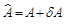the extremal problem can be formulated as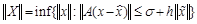(5)

From the computing point of view, the problem (5) is much simpler, than (4), as the constraint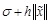in the right hand side can be obtained in advance. After noting that the perturbationis known in this case, we can formulate the following extremal problem: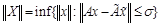(6)

The formulated variation problems are solved by the standard N Minimize[f,x] function which minimize f numerically with respect to x (Wolfram company).

4. Impementation

We define the ill-conditioned matrixin which the elements on the main diagonal are (+1), all lower diagonals are zeros, and the diagonals above have elements (-1):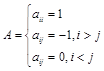In the Mathematica language 10.1 such matrix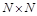is defined as: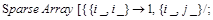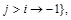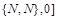.

In spite of the determinant value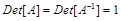, calculating the inverse matrix for N=512 becomes impossible because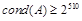. If we perturb A by adding a random matrixwith an amplitude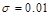, the inverse matrix can be calculated. The resulting solution from Linear Solve is shown in Fig. 2а.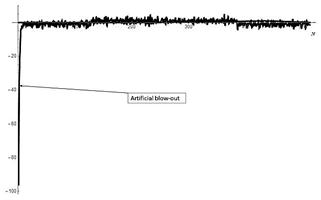Fig. 2a. The classical "solution" from intentionally perturbed system.

This solution coincides with its analytical model (square-wave pulse) in the majority of points, but it contains an essential error in the left hand side. If such classical solution doesn't satisfy the researcher, it can be further filtered by solving a varitional problem (5) or (6). The final solution in Fig. 2b. is close to its model in all points.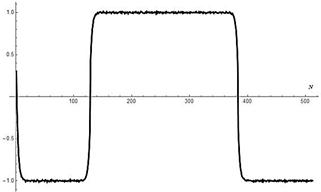Fig. 2b. Filtered variational waveform solution using the classical solution on Fig.2a.

Note: In this example it is possible to compare the classical and extremal solutions, but for huge matrices the variation method demands expensive computing efforts.

5. Well-Conditioned Systems

To our knowledge, the question about finding well-founded solution estimates of well-conditioned systems in the presence of noise in the coefficients is insufficiently studied in the literature on such systems. We may also not expect to reduce the condition number for well-conditioned systems of a big order under noise perturbation On the contrary, there is high probability that the condition number of a noisy matrix will grow with growing noise amplitude and the dimension of system, as the norm of a random matrix grows. If the matrix of A is non-singular and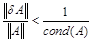(7)

then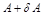is also non-singular (the theorem 2.3.1 ). For such systems the relative error of the solution at exact right right hand sideis calculated according to a known inequality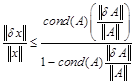(8)

For an example let us consider Toeplitz matrix of dimension N=1000x1000 with cond = 696745 with the matrix A perturbed with noise amplitude by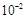. It is easy to check a condition of the above-mentioned theorem and an inequality (8). Value of the right part of (8) shows slow growth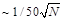depending on the dimension of system.

If to consider the effect of noise on the condition number at N=1000, we see that the system with well-conditioned Toeplitz matrix becomes ill-conditioned. Also observe exponential growth of the condition number of the matrixthough value of condition number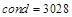of a random matrix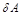is small compared to the noisy matrix. One can notice violation of estimate (8) if the noise amplitude is more than h= 0.048 and inequality (7) is not valid. Then the problem of solving of the system may be interpreted as incorrect or it can be also solved by a variational method. Modeling on a grid N=500 showed reduction of error in the classical solution, with the subsequent 10 time improvement if the variation method is further applied.

6. Conclusion

In this paper, we have analyzed the ill-posed problems from classical and variational point of view. For a solution of ill-posed systems there are still no methods available that could be considered as the best or the final. The purpose of the current work was to create a method for solution of systems that does not take into account the study on conditionality.

The two-step method proposed in this paper allows to get the numerical solution, and it has many advantages over the existing methods. If the algebraic system is well-posed, there is no sense to apply the time consuming variation method, which demands expensive computing resources. The influence of approximate coefficients of a matrix on the solution error in this case is minimal.

With ill-posed systems the situation is opposite, since the classical numerical methods are not applicable for such systems. If the classical solution is distorted and irregular, but the residual is small, it can be used as a source of a priori information to get the variational solution.

The proposed forced noise contamination of matrices opens a new way to combine different classical algorithms and variation methods for ill-posed problems (Fig. 3).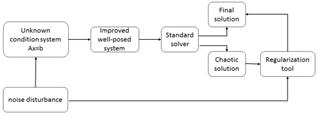Fig. 3. Connection between classical and variational methods.

References

1. A. Tikhonov and V. Arsenin, Solutions of ill-posed problems. Winston, Washington, DC(1977).
2. A.N. Tikhonov, A. S. Leonov, A. G. Yagola,Nonlinear Ill-Posed Problems (Applied Mathematical Sciences),Springer; Softcover reprint of the original 1st ed. 1998 edition (February 7, 2014).
3. Ivanov, Valentin K., Vladimir V. Vasin, and Vitalii P. Tanana. Theory of linear ill-posed problems and its applications. Vol. 36. Walter de Gruyter, 2002.
4. N. N. Kalitkin,L. F. Yuhno,L. V. Kuz’mina, Quantitative criterion of conditioning for systems of linear algebraic equations,Mathematical Models and Computer SimulationsOctober 2011, Volume 3,Issue 5, pp 541-556
5. Hansen, P. C. (2007). Regularization tools version 4.0 for Matlab 7.3. Numerical Algorithms, 46(2), 189-194.
6. A. Bakushinsky and A. Goncharsky, Ill-posed problems: theory and applications. Springer Netherlands (October 9, 2012).
7. Terence Tao and Van Vu. Smooth analysis of the condition number and the least singular value. Mathematics of computation ,Volume 79, Number 272, October 2010, Pages 2333–2352
8. A. Edelman. Eigenvalues and condition numbers of random matrices. SIAM j. Matrix Anal. Appl., Vol.9, No. 4, October, 1988, Pages 543- 560.
9. David S. Watkins. Fundamentals of Matrix Computations, Third EditionJohn Wiley and Sons, July 2010, 644 pp.

 Contents 1. 2. 3. 4. 5. 6.
Article ToolsAbstractPDF(720K)